NCERT Solutions for Class 7 Maths Chapter 6 The Triangle and its Properties Ex 6.3 are part of NCERT Solutions for Class 7 Maths. Here we have given NCERT Solutions for Class 7 Maths Chapter 6 The Triangle and its Properties Ex 6.3.

 Board CBSE Textbook NCERT Class Class 7 Subject Maths Chapter Chapter 6 Chapter Name The Triangle and its Properties Exercise Ex 6.3 Number of Questions Solved 2 Category NCERT Solutions

## NCERT Solutions for Class 7 Maths Chapter 6 The Triangle and its Properties Ex 6.3

Question 1.
Find the value of the unknown x in the following diagrams: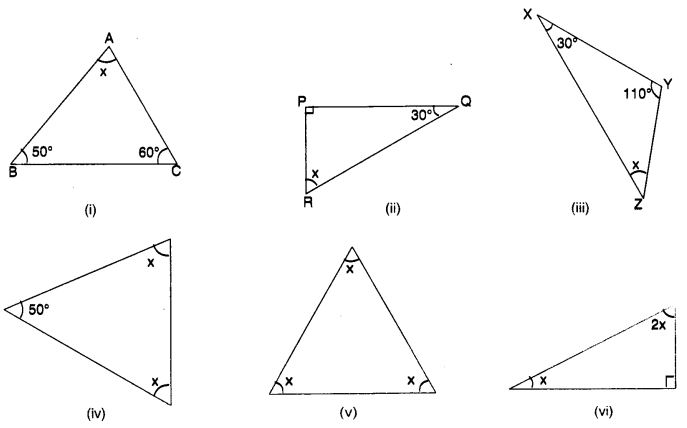Solution: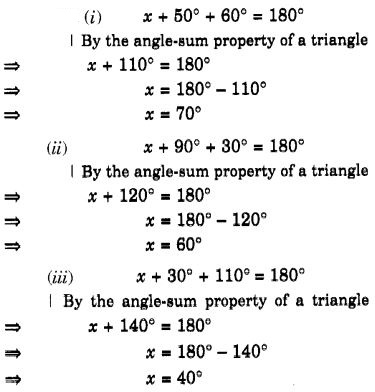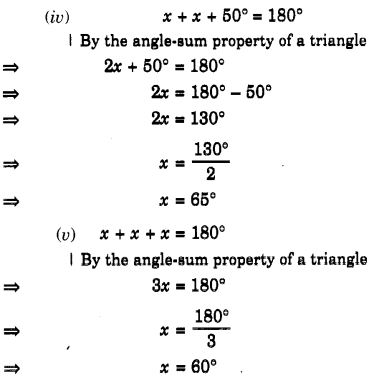Question 2.
Find the values of the unknowns x and y in the following diagrams: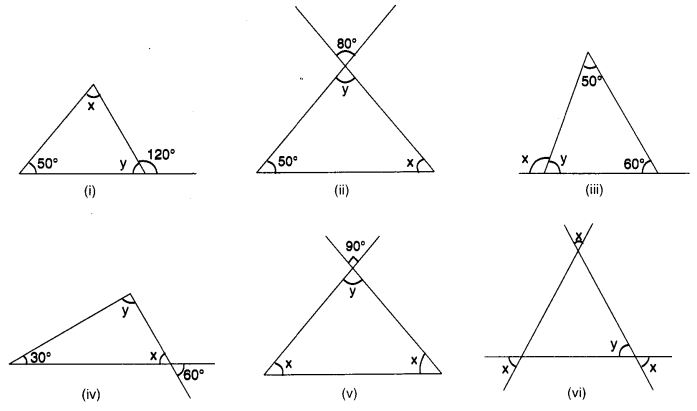Solution: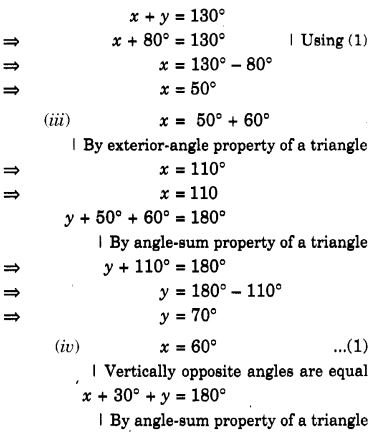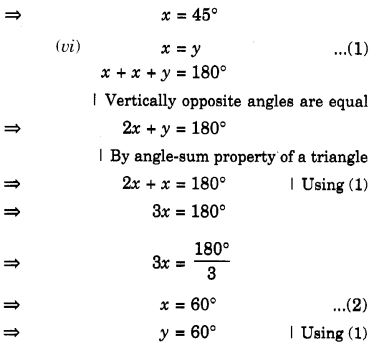We hope the NCERT Solutions for Class 7 Maths Chapter 6 The Triangle and its Properties Ex 6.3 help you. If you have any query regarding NCERT Solutions for Class 7 Maths Chapter 6 The Triangle and its Properties Ex 6.3, drop a comment below and we will get back to you at the earliest.